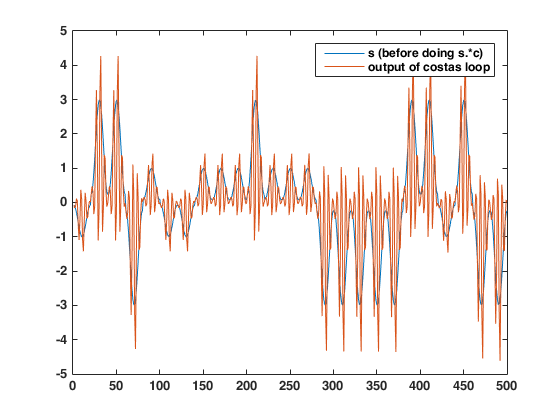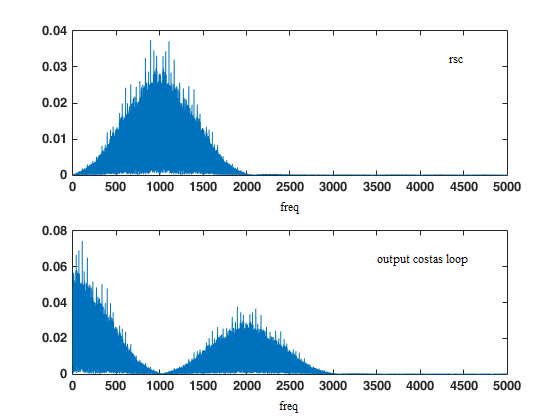## Having troubles implementing Costas loop for 4-PAMStarted by 4 years ago114 views
Carrier recovery is discussed in Chapter 10 in "Telecommunication Breakdown" by Johnson and Sethares. I've been running the code they provide and I get the same result when I plot "theta" to see how it changes overtime and it looks the same as the screenshot in the book. But I tried to look at the output of the costas lop (which is not discussed nor plotted in the chapter, not sure why) and I don't seem to be getting very good results. Here's the code from the book (made slight modifications to get the output and also replaced the pam() function with pammod() cause I didn't find such function in the book and I don't have the CD-ROM):
N=10000; M=20; Ts=.0001;
time=Ts*(N*M-1); t=0:Ts:time;
m=pammod(randi([0 3],1,N),4);
mup=zeros(1,N*M); mup(1:M:end)=m;
ps=hamming(M);
s=filter(ps,1,mup);
f0=1000; phoff=-1.0;
c=cos(2*pi*f0*t+phoff);
rsc=s.*c;
rlc=(s+1).*c;

fftrlc=fft(rlc);
[mx,imax]=max(abs(fftrlc(1:end/2)));
ssf=(0:length(t))/(Ts*length(t));
freqL=ssf(imax)
phaseL=angle(fftrlc(imax))

%costasr
r=rsc;
fl=500; ff=[0 .01 .02 1]; fa=[1 1 0 0];
h=remez(fl,ff,fa);
mu=.003;
fc=1000;
theta=zeros(1,length(t)); theta(1)=0;
zs=zeros(1,fl+1); zc=zeros(1,fl+1);
output=zeros(1,length(t));
for k=1:length(t)-1
output(k) = 2*r(k)*cos(2*pi*fc*t(k)+theta(k));
zs=[zs(2:fl+1), 2*r(k)*sin(2*pi*fc*t(k)+theta(k))];
zc=[zc(2:fl+1), output(k)];
lpfs=fliplr(h)*zs'; lpfc=fliplr(h)*zc';
theta(k+1)=theta(k)-mu*lpfs*lpfc;
end

So I decided to plot output vs s and this is what I got:If I understood the theory about Costas loop, if the input is $$s(t)=m(t)*cos(2*pi*f_0*t+theta_i)$$, if the loop worked fine, at the output I should have $$0.5∗m(t)cos(theta_i-theta_o)=0.5∗m(t)cos(theta_e)$$ -> for theta_e ~ 0 -> $$0.5∗m(t)$$ but as you can see, there's something else besides m(t). Why is this happening? I tried plotting the FFT of the rsc and the output, and as you can see, there is some info at around 2000 Hz that shouldn't be there, only the baseband should:Also,  I've tried a different way of implementing it http://read.pudn.com/downloads80/doc/project/307210/CarrierRecoveryUsingaSecondOrderCostasLoop.pdf and I keep getting the same result, despite the author getting it to work properly